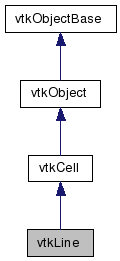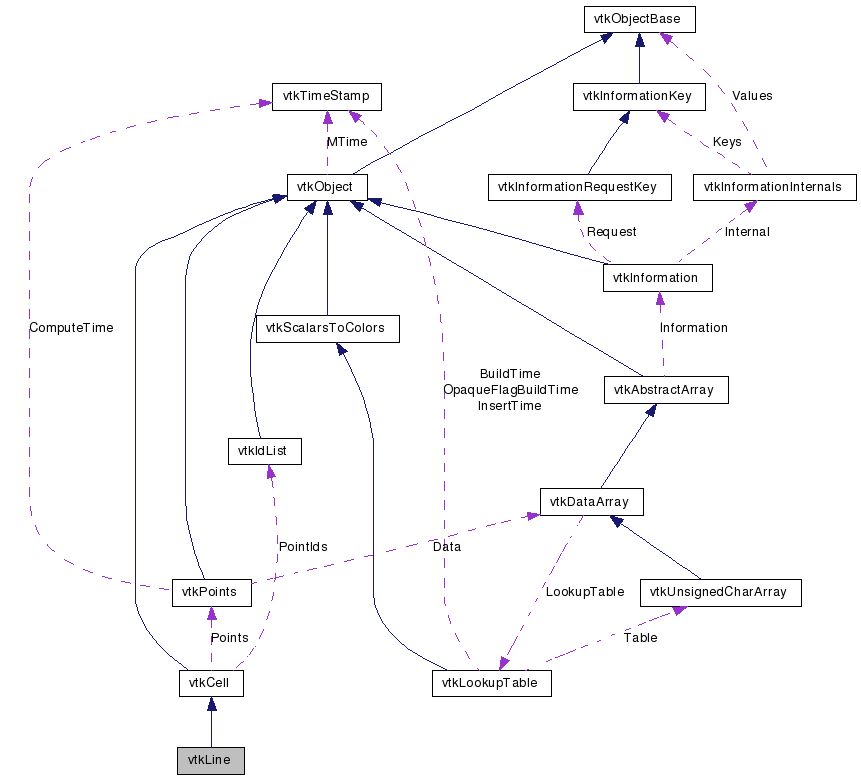vtkLine Class Reference

#include <vtkLine.h>

Inheritance diagram for vtkLine:[legend]
Collaboration diagram for vtkLine:[legend]

Detailed Description

cell represents a 1D line

vtkLine is a concrete implementation of vtkCell to represent a 1D line.

Tests:
vtkLine (Tests)

Definition at line 29 of file vtkLine.h.

static void InterpolationFunctions (double pcoords, double weights)
static void InterpolationDerivs (double pcoords, double derivs)
virtual void InterpolateFunctions (double pcoords, double weights)
virtual void InterpolateDerivs (double pcoords, double derivs)

Public Types

typedef vtkCell Superclass

Public Member Functions

virtual const char * GetClassName ()
virtual int IsA (const char *type)
void PrintSelf (ostream &os, vtkIndent indent)
int GetParametricCenter (double pcoords)
int GetCellType ()
int GetCellDimension ()
int GetNumberOfEdges ()
int GetNumberOfFaces ()
vtkCellGetEdge (int)
vtkCellGetFace (int)
int CellBoundary (int subId, double pcoords, vtkIdList *pts)
void Contour (double value, vtkDataArray *cellScalars, vtkPointLocator *locator, vtkCellArray *verts, vtkCellArray *lines, vtkCellArray *polys, vtkPointData *inPd, vtkPointData *outPd, vtkCellData *inCd, vtkIdType cellId, vtkCellData *outCd)
int EvaluatePosition (double x, double *closestPoint, int &subId, double pcoords, double &dist2, double *weights)
void EvaluateLocation (int &subId, double pcoords, double x, double *weights)
int Triangulate (int index, vtkIdList *ptIds, vtkPoints *pts)
void Derivatives (int subId, double pcoords, double *values, int dim, double *derivs)
virtual double * GetParametricCoords ()
void Clip (double value, vtkDataArray *cellScalars, vtkPointLocator *locator, vtkCellArray *lines, vtkPointData *inPd, vtkPointData *outPd, vtkCellData *inCd, vtkIdType cellId, vtkCellData *outCd, int insideOut)
int IntersectWithLine (double p1, double p2, double tol, double &t, double x, double pcoords, int &subId)

Static Public Member Functions

static vtkLineNew ()
static int IsTypeOf (const char *type)
static vtkLineSafeDownCast (vtkObject *o)
static double DistanceToLine (double x, double p1, double p2)
static int Intersection (double p1, double p2, double x1, double x2, double &u, double &v)
static double DistanceToLine (double x, double p1, double p2, double &t, double closestPoint)
static double DistanceBetweenLines (double l0, double l1, double m0, double m1, double closestPt1, double closestPt2, double &t1, double &t2)
static double DistanceBetweenLineSegments (double l0, double l1, double m0, double m1, double closestPt1, double closestPt2, double &t1, double &t2)

vtkLine ()
~vtkLine ()

Member Typedef Documentation

 typedef vtkCell vtkLine::Superclass

Reimplemented from vtkCell.

Definition at line 33 of file vtkLine.h.

Constructor & Destructor Documentation

 vtkLine::vtkLine ( ) [protected]

 vtkLine::~vtkLine ( ) [inline, protected]

Definition at line 160 of file vtkLine.h.

Member Function Documentation

 static vtkLine* vtkLine::New ( ) [static]

Create an object with Debug turned off, modified time initialized to zero, and reference counting on.

Reimplemented from vtkObject.

 virtual const char* vtkLine::GetClassName ( ) [virtual]

Reimplemented from vtkCell.

 static int vtkLine::IsTypeOf ( const char * name ) [static]

Return 1 if this class type is the same type of (or a subclass of) the named class. Returns 0 otherwise. This method works in combination with vtkTypeRevisionMacro found in vtkSetGet.h.

Reimplemented from vtkCell.

 virtual int vtkLine::IsA ( const char * name ) [virtual]

Return 1 if this class is the same type of (or a subclass of) the named class. Returns 0 otherwise. This method works in combination with vtkTypeRevisionMacro found in vtkSetGet.h.

Reimplemented from vtkCell.

 static vtkLine* vtkLine::SafeDownCast ( vtkObject * o ) [static]

Reimplemented from vtkCell.

 void vtkLine::PrintSelf ( ostream & os, vtkIndent indent ) [virtual]

Methods invoked by print to print information about the object including superclasses. Typically not called by the user (use Print() instead) but used in the hierarchical print process to combine the output of several classes.

Reimplemented from vtkCell.

 int vtkLine::GetCellType ( ) [inline, virtual]

See the vtkCell API for descriptions of these methods.

Implements vtkCell.

Definition at line 38 of file vtkLine.h.

 int vtkLine::GetCellDimension ( ) [inline, virtual]

See the vtkCell API for descriptions of these methods.

Implements vtkCell.

Definition at line 39 of file vtkLine.h.

 int vtkLine::GetNumberOfEdges ( ) [inline, virtual]

See the vtkCell API for descriptions of these methods.

Implements vtkCell.

Definition at line 40 of file vtkLine.h.

 int vtkLine::GetNumberOfFaces ( ) [inline, virtual]

See the vtkCell API for descriptions of these methods.

Implements vtkCell.

Definition at line 41 of file vtkLine.h.

 vtkCell* vtkLine::GetEdge ( int ) [inline, virtual]

See the vtkCell API for descriptions of these methods.

Implements vtkCell.

Definition at line 42 of file vtkLine.h.

 vtkCell* vtkLine::GetFace ( int ) [inline, virtual]

See the vtkCell API for descriptions of these methods.

Implements vtkCell.

Definition at line 43 of file vtkLine.h.

 int vtkLine::CellBoundary ( int subId, double pcoords, vtkIdList * pts ) [virtual]

See the vtkCell API for descriptions of these methods.

Implements vtkCell.

 void vtkLine::Contour ( double value, vtkDataArray * cellScalars, vtkPointLocator * locator, vtkCellArray * verts, vtkCellArray * lines, vtkCellArray * polys, vtkPointData * inPd, vtkPointData * outPd, vtkCellData * inCd, vtkIdType cellId, vtkCellData * outCd ) [virtual]

See the vtkCell API for descriptions of these methods.

Implements vtkCell.

 int vtkLine::EvaluatePosition ( double x, double * closestPoint, int & subId, double pcoords, double & dist2, double * weights ) [virtual]

See the vtkCell API for descriptions of these methods.

Implements vtkCell.

 void vtkLine::EvaluateLocation ( int & subId, double pcoords, double x, double * weights ) [virtual]

See the vtkCell API for descriptions of these methods.

Implements vtkCell.

 int vtkLine::Triangulate ( int index, vtkIdList * ptIds, vtkPoints * pts ) [virtual]

See the vtkCell API for descriptions of these methods.

Implements vtkCell.

 void vtkLine::Derivatives ( int subId, double pcoords, double * values, int dim, double * derivs ) [virtual]

See the vtkCell API for descriptions of these methods.

Implements vtkCell.

 virtual double* vtkLine::GetParametricCoords ( ) [virtual]

See the vtkCell API for descriptions of these methods.

Reimplemented from vtkCell.

 void vtkLine::Clip ( double value, vtkDataArray * cellScalars, vtkPointLocator * locator, vtkCellArray * lines, vtkPointData * inPd, vtkPointData * outPd, vtkCellData * inCd, vtkIdType cellId, vtkCellData * outCd, int insideOut ) [virtual]

Clip this line using scalar value provided. Like contouring, except that it cuts the line to produce other lines.

Implements vtkCell.

 int vtkLine::GetParametricCenter ( double pcoords ) [inline, virtual]

Return the center of the triangle in parametric coordinates.

Reimplemented from vtkCell.

Definition at line 168 of file vtkLine.h.

 int vtkLine::IntersectWithLine ( double p1, double p2, double tol, double & t, double x, double pcoords, int & subId ) [virtual]

Line-line intersection. Intersection has to occur within [0,1] parametric coordinates and with specified tolerance.

Implements vtkCell.

 static int vtkLine::Intersection ( double p1, double p2, double x1, double x2, double & u, double & v ) [static]

Performs intersection of two finite 3D lines. An intersection is found if the projection of the two lines onto the plane perpendicular to the cross product of the two lines intersect. The parameters (u,v) are the parametric coordinates of the lines at the position of closest approach.

 static double vtkLine::DistanceToLine ( double x, double p1, double p2, double & t, double closestPoint ) [static]

Compute the distance of a point x to a finite line (p1,p2). The method computes the parametric coordinate t and the point location on the line. Note that t is unconstrained (i.e., it may lie outside the range [0,1]) but the closest point will lie within the finite line [p1,p2]. Also, the method returns the distance squared between x and the line (p1,p2).

 static double vtkLine::DistanceToLine ( double x, double p1, double p2 ) [static]

Determine the distance of the current vertex to the edge defined by the vertices provided. Returns distance squared. Note: line is assumed infinite in extent.

 static double vtkLine::DistanceBetweenLines ( double l0, double l1, double m0, double m1, double closestPt1, double closestPt2, double & t1, double & t2 ) [static]

Computes the shortest distance squared between two infinite lines, each defined by a pair of points (l0,l1) and (m0,m1). Upon return, the closest points on the two line segments will be stored in closestPt1 and closestPt2. Their parametric coords (-inf <= t0, t1 <= inf) will be stored in t0 and t1. The return value is the shortest distance squared between the two line-segments.

 static double vtkLine::DistanceBetweenLineSegments ( double l0, double l1, double m0, double m1, double closestPt1, double closestPt2, double & t1, double & t2 ) [static]

Computes the shortest distance squared between two finite line segments defined by their end points (l0,l1) and (m0,m1). Upon return, the closest points on the two line segments will be stored in closestPt1 and closestPt2. Their parametric coords (0 <= t0, t1 <= 1) will be stored in t0 and t1. The return value is the shortest distance squared between the two line-segments.

 static void vtkLine::InterpolationFunctions ( double pcoords, double weights ) [static]

Deprecated:
Replaced by vtkLine::InterpolateFunctions as of VTK 5.2

 static void vtkLine::InterpolationDerivs ( double pcoords, double derivs ) [static]

Deprecated:
Replaced by vtkLine::InterpolateFunctions as of VTK 5.2

 virtual void vtkLine::InterpolateFunctions ( double pcoords, double weights ) [inline, virtual]

Deprecated:
Replaced by vtkLine::InterpolateFunctions as of VTK 5.2

Definition at line 148 of file vtkLine.h.

 virtual void vtkLine::InterpolateDerivs ( double pcoords, double derivs ) [inline, virtual]

Deprecated:
Replaced by vtkLine::InterpolateFunctions as of VTK 5.2

Definition at line 152 of file vtkLine.h.

The documentation for this class was generated from the following file:

Generated on Wed Jun 3 19:15:29 2009 for VTK by1.5.6